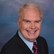# Math Solutions: Wet Well Flow Rate CalculationsBy Daniel Theobald, "Wastewater Dan"

Water Online’s “Math Solutions” series, presented by wastewater consultant and trainer Dan Theobald (“Wastewater Dan”), instructs operators on a variety of calculations necessary for plant operations and operator certification. Here Dan tackles wet well calculations, with a bonus calculation for determining flow in cubic feet.

Calculate flow entering wet well – presented in three installments:

1.) Calculate Gallons Of Wet Well Effluent:

2.) Calculate Gallons Of Wet Well Level Increase:

3.) Calculate GPM Of Wet Well Influent:

Calculate flow rate in cubic feet per second:

Complete playlist of Wastewater Dan’s Math Solutions

This is the ‘Wet Well Flow Rate Calculations' presentation in my series of “Math Solutions.” If you have specific wastewater math queries, please submit a question.